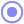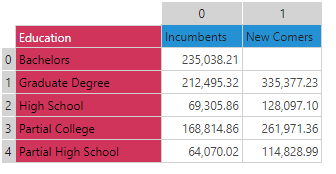#Loop (PQL)

Iterates and returns a combined result of all the looped text strings.

• Returned Output: Text
• Library: PQL \ Common \ Logical
• Version: 2020.10.000
• Compatibility:
• Can be combined with and other PQL function throughout the application.
• It CANNOT be used with MDX or VBA functions. But it can be used on MDX-based content in other parts of the application.

#### Syntax

Loop( <Text> , )

* Click on the function's arguments above for more details on the input values.

• The function will iterate or loop over the supplied text and produce a concatenated text string as the result. This is useful for building dynamic lists of items.
• The supplied text can be static or any functional representation.
• Loop Iterations is a value representing the number of times the loop logic should iterate.
• This is a single loop - operating over a single index only
##### Using the Loop Index

The loop function has an internal counter called the "index". It will resolve to a positive whole number starting at 0 and incrementing by 1 for each loop. You can use this index to drive logic in your formulas as you dynamically create text.

##### Different Function types

Also see LoopVector.

#### Examples

This example returns "test test test" - simply looping 3 times and concatenating the word "test" each time.

Loop ("test ", 3)

This example uses the following grid:Notice that the "index" is used to automatically change the row position in the data function. It starts at 0 and changes 3 times (0,1,2)

Loop (Bullet("The value is " + data(index,0)) , 3)

The result here is of this logic is:

• The value is 235038.21
• The value is 212495.32
• The value is 69305.86